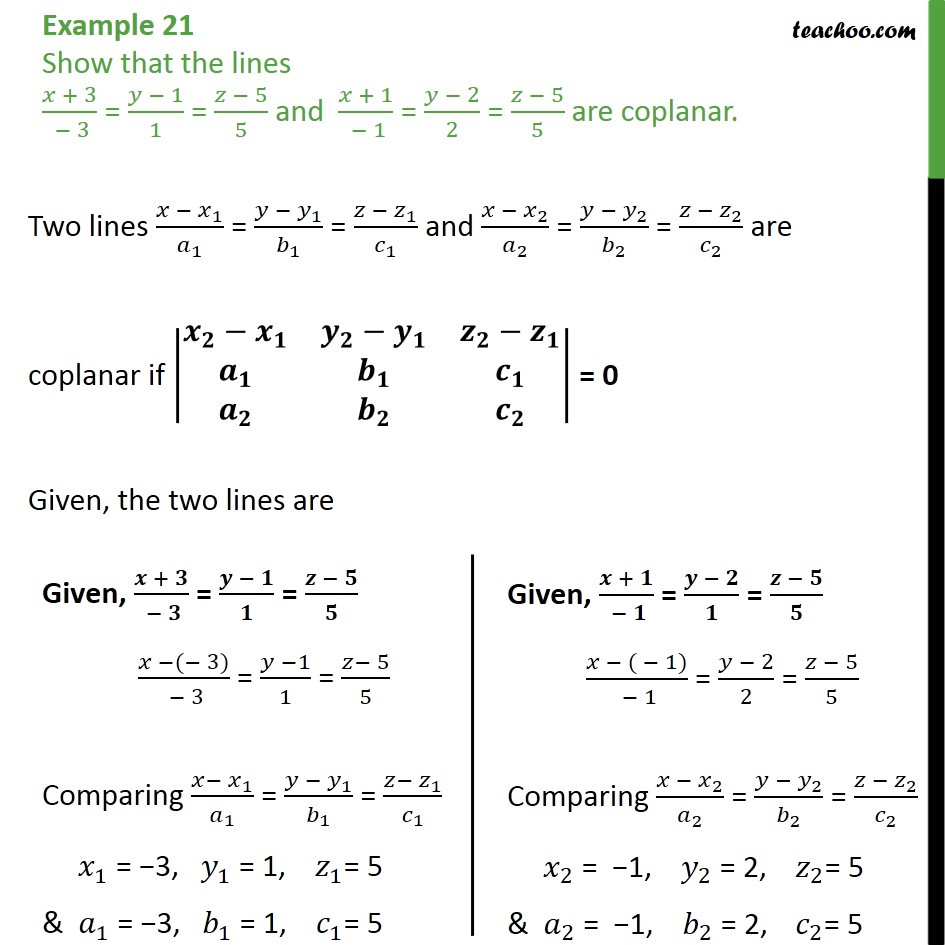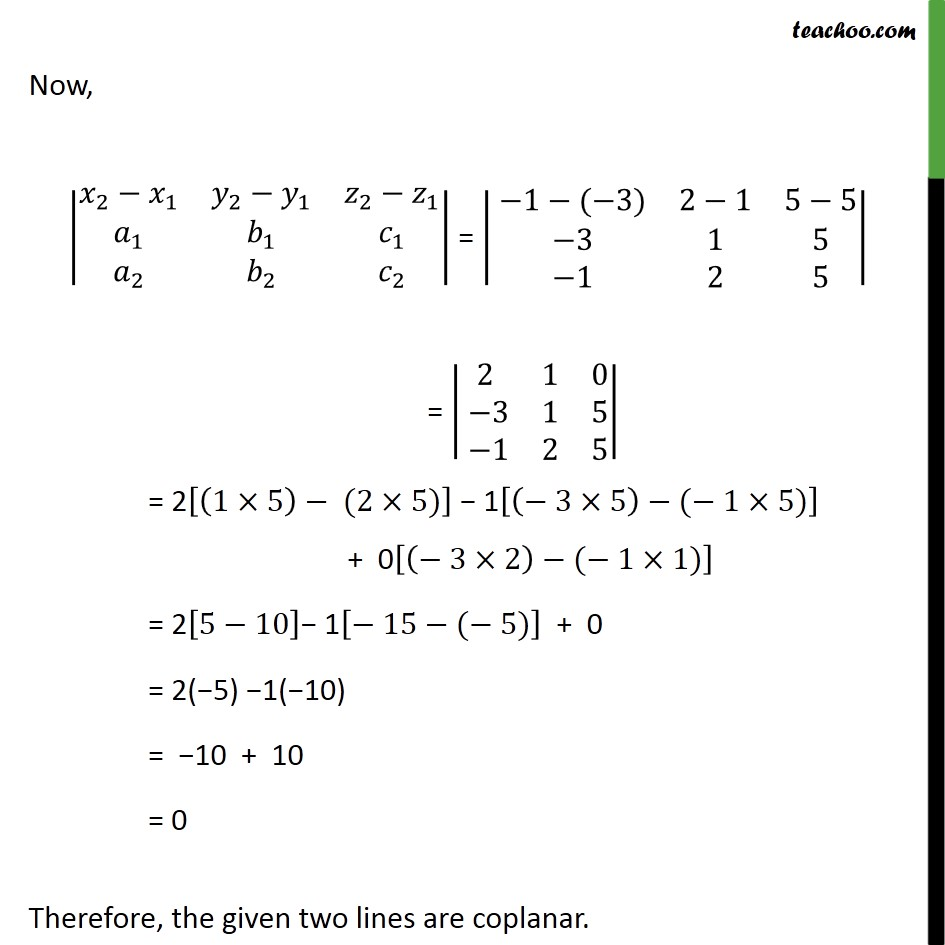1. Chapter 11 Class 12 Three Dimensional Geometry
2. Concept wise
3. Coplanarity of 2 lines

Transcript

Example 21 Show that the lines + 3 3 = 1 1 = 5 5 and + 1 1 = 2 2 = 5 5 are coplanar. Two lines 1 1 = 1 1 = 1 1 and 2 2 = 2 2 = 2 2 are coplanar if = 0 Given, the two lines are Now, 2 1 2 1 2 1 1 1 1 2 2 2 = 1 ( 3) 2 1 5 5 3 1 5 1 2 5 = 2 1 0 3 1 5 1 2 5 = 2 1 5 (2 5) 1 3 5 ( 1 5) + 0 3 2 ( 1 1) = 2 5 10 1 15 ( 5) + 0 = 2( 5) 1( 10) = 10 + 10 = 0 Therefore, the given two lines are coplanar.

Coplanarity of 2 lines

About the AuthorDavneet Singh
Davneet Singh is a graduate from Indian Institute of Technology, Kanpur. He has been teaching from the past 9 years. He provides courses for Maths and Science at Teachoo.